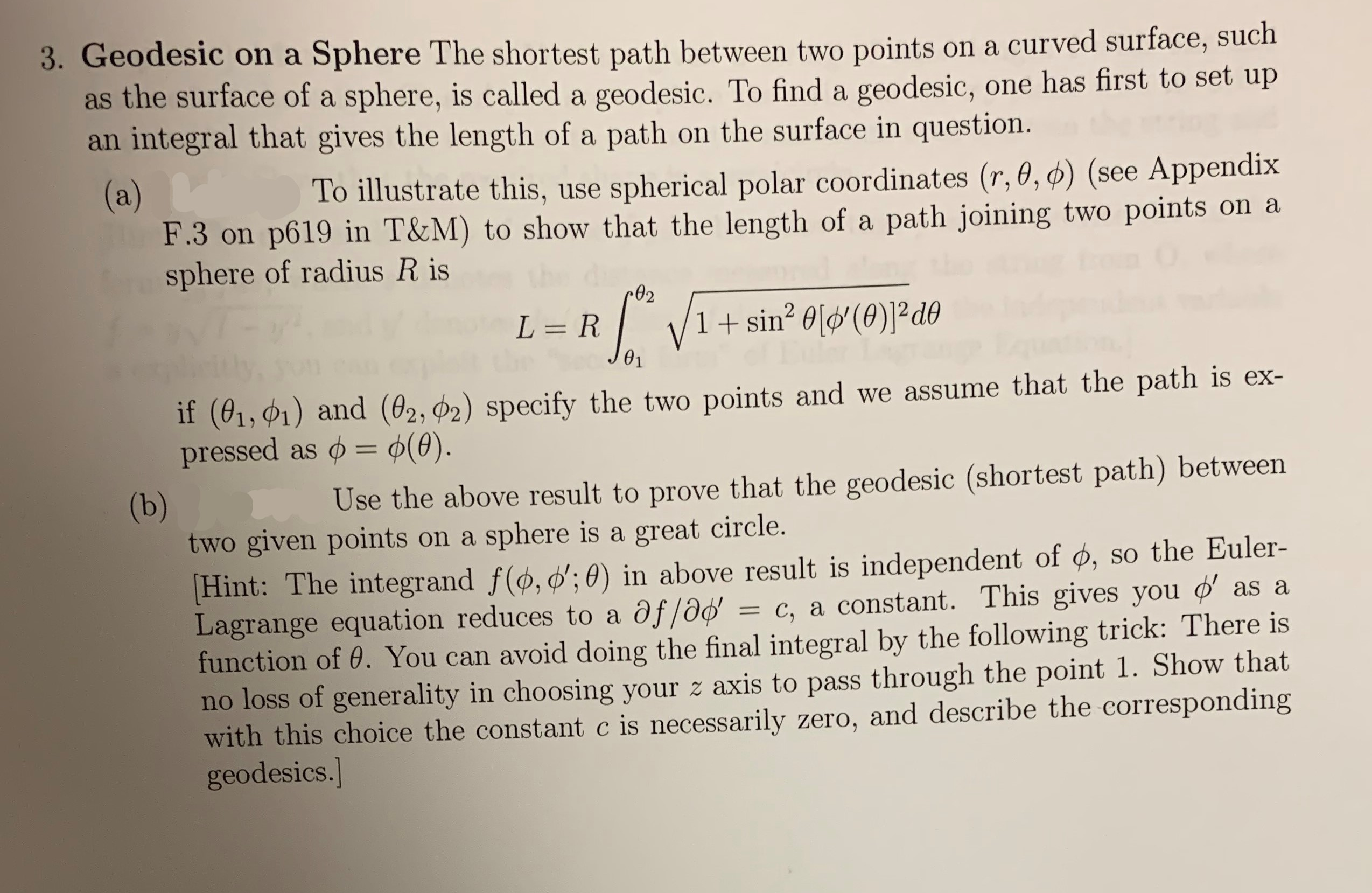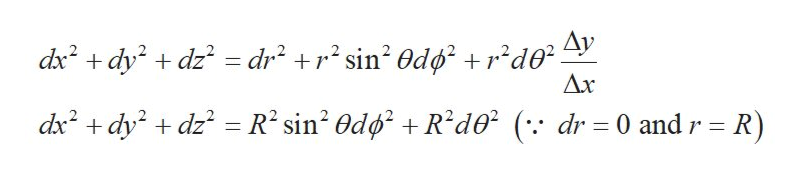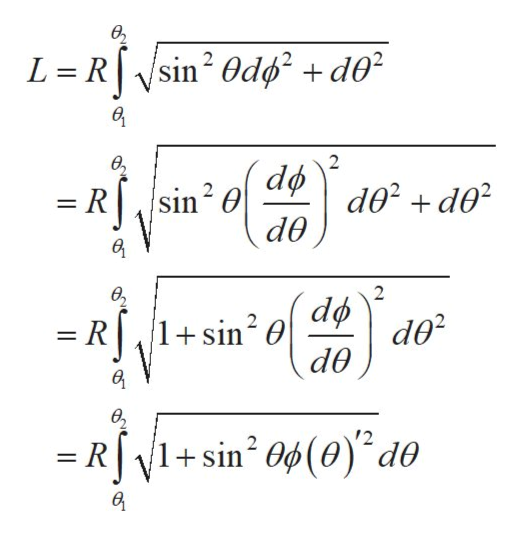# 3. Geodesic on a Sphere The shortest path between two points on a curved surface, suchas the surface of a sphere, is called a geodesic. To find a geodesic, one has first to set upan integral that gives the length of a path on the surface in question.(a)F.3 on p619 in T&M) to show that the length of a path joining two points on asphere of radius R isTo illustrate this, use spherical polar coordinates (r, 0, ø) (see Appendix1+sin2 0l(0)2 deL=R01if (01,1) and (02, P2) specify the two points and we assume that the path is ex-pressed as = (0).(b)two given points on a sphere is a great circle.Use the above result to prove that the geodesic (shortest path) betweenHint: The integrand f(o, ;0) in above result is independent of ø, so the Euler-Lagrange equation reduces to a af/od = c, a constant. This gives you as afunction of 0. You can avoid doing the final integral by the following trick: There isno loss of generality in choosing your z axis to pass through the point 1. Show thatwith this choice the constant c is necessarily zero, and describe the correspondinggeodesics.

Questionhelp_outlineImage Transcriptionclose3. Geodesic on a Sphere The shortest path between two points on a curved surface, such as the surface of a sphere, is called a geodesic. To find a geodesic, one has first to set up an integral that gives the length of a path on the surface in question. (a) F.3 on p619 in T&M) to show that the length of a path joining two points on a sphere of radius R is To illustrate this, use spherical polar coordinates (r, 0, ø) (see Appendix 1+sin2 0l(0)2 de L=R 01 if (01,1) and (02, P2) specify the two points and we assume that the path is ex- pressed as = (0). (b) two given points on a sphere is a great circle. Use the above result to prove that the geodesic (shortest path) between Hint: The integrand f(o, ;0) in above result is independent of ø, so the Euler- Lagrange equation reduces to a af/od = c, a constant. This gives you as a function of 0. You can avoid doing the final integral by the following trick: There is no loss of generality in choosing your z axis to pass through the point 1. Show that with this choice the constant c is necessarily zero, and describe the corresponding geodesics. fullscreen
check_circleExpert Solution
Step 1

(a)Write the formula for the length(L) of a curve in 3-dimension.

Step 2

Use the spherical coordinate(r,θ,ϕ) and find the value of dx2+ dy2+ dz2 as,help_outlineImage Transcriptionclose=dr2 2 sin2 Odrd0* ^V Ax dxdydz dx2 dydz R2 sin2 Od Rd0 ( dr 0 and r = R) .. fullscreen
Step 3

Plug the value of dx2+ dy2+ dz2 in the equation (1) and solve for ...help_outlineImage Transcriptioncloseе, L Rsin2 Odd d0 2 ө, d02 d0 d0 = R,/sin2 = R,/1+sin2 0 de в в, =RV1+sin 04(0)° de 2 в fullscreen

### Want to see the full answer?

See Solution

#### Want to see this answer and more?

Solutions are written by subject experts who are available 24/7. Questions are typically answered within 1 hour*

See Solution
*Response times may vary by subject and question
Tagged in

### Science